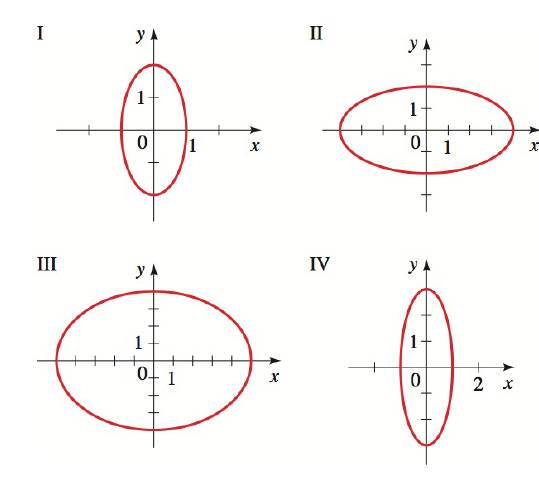Chapter 12.2, Problem 7E### Algebra and Trigonometry (MindTap ...

4th Edition
James Stewart + 2 others
ISBN: 9781305071742

#### Solutions

Chapter
Section### Algebra and Trigonometry (MindTap ...

4th Edition
James Stewart + 2 others
ISBN: 9781305071742
Textbook Problem

# SKILLS5-8 Graphs of Ellipses Match the equation with the graphs labeled I-IV. Give reasons for your answers. 4 x 2 + y 2 = 4To determine

To find:

The matched graph for ellipse 4x2+y2=4.

Explanation

Given:

The equation of an ellipse is 4x2+y2=4.

Approach:

The basic equation for an ellipse with a horizontal major axis which is used is as follows,

x2b2+y2a2=1

Here, a and b are constant values.

Expression to find vertices is,

V=(0,±a)

Expression to find Foci is,

F=(0,±c),

Here, c2=a2b2

Plot the graph for the given ellipse and compare it with the given graph. As if the plotted graph and given graph have similarity then that graph is the perfect match for the given equation of the ellipse.

Calculation:

Consider the given equation of the ellipse,

4x2+y2=44x24+y24=1x21+y24=1 ……(1)

Compare the above equation with the basic equation for an ellipse and find the vertices as follows,

a2=4a=±2

And,

b2=1b=±1

Find the vertices,

### Still sussing out bartleby?

Check out a sample textbook solution.

See a sample solution

#### The Solution to Your Study Problems

Bartleby provides explanations to thousands of textbook problems written by our experts, many with advanced degrees!

Get Started